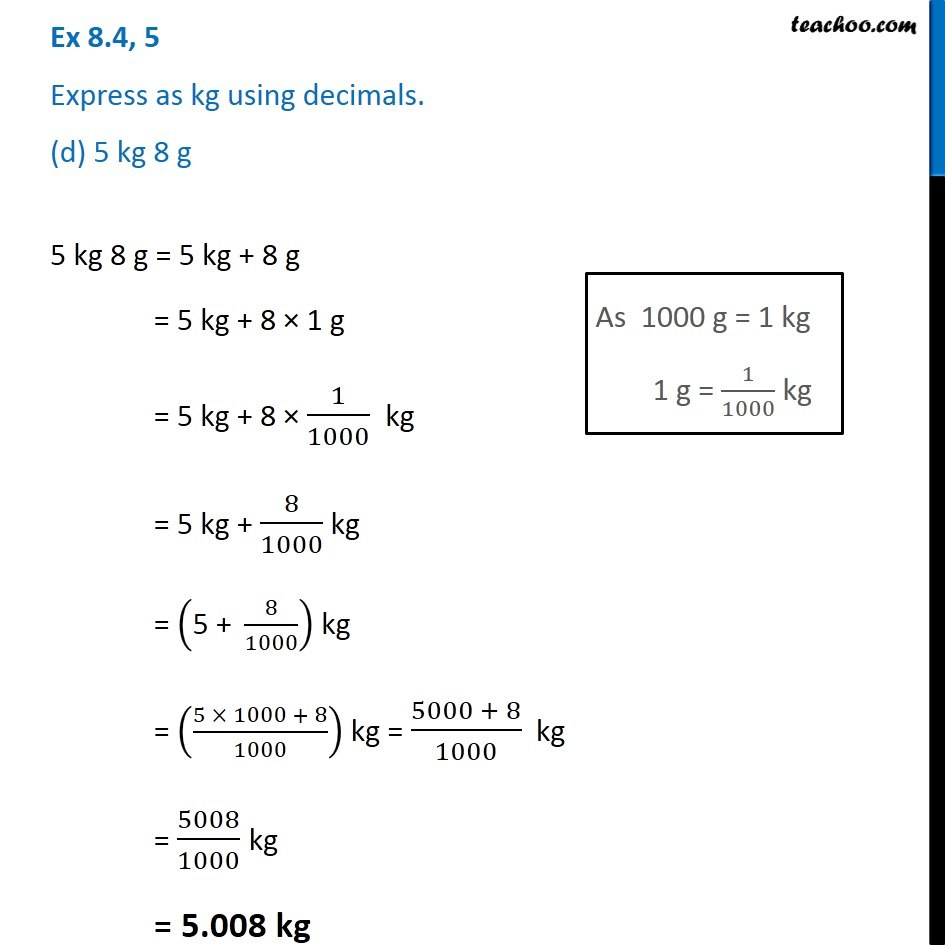1. Chapter 8 Class 6 Decimals
2. Serial order wise
3. Ex 8.4

Transcript

Ex 8.4, 5 Express as kg using decimals. (d) 5 kg 8 g 5 kg 8 g = 5 kg + 8 g = 5 kg + 8 × 1 g = 5 kg + 8 × 1/1000 kg = 5 kg + 8/1000 kg = ("5 + " 8/1000) kg = ((5 × 1000 + 8)/1000) kg = (5000 + 8)/1000 kg = 5008/1000 kg = 5.008 kg